# Review — AdderNet: Do We Really Need Multiplications in Deep Learning? (Image Classification)

## Using Addition Instead of Multiplication for Convolution, Lower Latency Than the Conventional CNN

In this story, AdderNet: Do We Really Need Multiplications in Deep Learning?, (AdderNet), by Peking University, Huawei Noah’s Ark Lab, and The University of Sydney, is reviewed.

Do We Really Need Multiplications in Deep Learning?

In this paper:

• AdderNet, using additions, takes the l1-norm distance between filters and input feature as the output response.
• Compared with multiplications, additions are much cheaper and reduce the computation costs.

This is a paper in 2020 CVPR with over 20 citations. (Sik-Ho Tsang @ Medium)

# Outline

2. Other Concerning Issues: BN, Derivatives, Learning Rate
3. Experimental Results

## 1.1. Generalized Filters

• Generally, the output feature Y indicates the similarity between the filter and the input feature:
• where S is the similarity measure.

## 1.2. Standard Convolution Using Multiplication

• If cross-correlation is taken as the metric of distance, multiplication is used. It becomes convolution.

• If addition is used, l1 distance between the filter and the input feature is calculated:
• With the help of l1 distance, similarity between the filters and features can be efficiently computed.

Addition is much lower computational expensive than multiplication.

Intuitively, the above equation has a connection with template matching in computer vision, which aims to find the parts of an image that match the template.

# 2. Other Concerning Issues: BN, Derivatives, Learning Rate

## 2.1. Batch Normalization (BN)

• After addition, batch normalization (BN) is used to normalize Y to an appropriate range and all the activation functions used in conventional CNNs can then be used in the proposed AdderNets.
• Although the BN layer involves multiplications, its computational cost is significantly lower than that of the convolutional layers and can be omitted.
• (Will there be any BN just using addition in the future?)

## 2.2. Derivatives

• The derivative of l1-norm is not good for gradient descent. Thus, the derivative of l2-norm is considered:
• By utilizing the full-precision gradient, the filters can be updated precisely.
• To prevent gradients from exploding, the gradient of X is clipped to [-1,1].
• Then the partial derivative of output features Y with respect to the input features X is calculated as:
• where HT is HardTanh function:

• As shown in this table, the norms of gradients of filters in AdderNets are much smaller than that in CNNs, which could slow down the update of filters in AdderNets.
• An adaptive learning rate for different layers in AdderNets is used:
• where γ is a global learning rate of the whole neural network (e.g. for adder and BN layers), ΔL(Fl) is the gradient of the filter in layer l and αl is its corresponding local learning rate.
• The local learning rate can therefore be defined as:
• where k denotes the number of elements in Fl, and η is a hyper-parameter to control the learning rate of adder filters.

# 3. Experimental Results

## 3.1. MNIST

• LeNet-5-BN is trained.
• CNN achieves a 99.4% accuracy with 435K multiplications and 435K additions.
• By replacing the multiplications in convolution with additions, the proposed AdderNet achieves a 99.4% accuracy, which is the same as that of CNNs, with 870K additions and almost no multiplication.
• In fact, the theoretical latency of multiplications in CPUs is also larger than that of additions and subtractions.
• For example, in VIA Nano 2000 series, the latency of float multiplication and addition is 4 and 2, respectively. The AdderNet using LeNet-5 model will have 1.7M latency while CNN will have 2.6M latency in this CPU.

## 3.2. CIFAR

• Binary neural networks (BNN): It can use the XNOR operations to replace multiplications, it is also used for comparison.
• For VGG-small model, AdderNets achieve nearly the same results (93.72% in CIFAR-10 and 72.64% in CIFAR-100) with CNNs (93.80% in CIFAR-10 and 72.73% in CIFAR-100) with no multiplication.
• Although the model size of BNN is much smaller than those of AdderNet and CNN, its accuracies are much lower (89.80% in CIFAR-10 and 65.41% in CIFAR-100).
• As for the ResNet-20, CNNs achieve the highest accuracy (i.e. 92.25% in CIFAR-10 and 68.14% in CIFAR-100) but with a large number of multiplications (41.17M).
• The proposed AdderNets achieve a 91.84% accuracy in CIFAR-10 and a 67.60% accuracy in CIFAR-100 without multiplications, which is comparable with CNNs.
• In contrast, the BNNs only achieve 84.87% and 54.14% accuracies in CIFAR-10 and CIFAR-100.
• The results in ResNet-32 also suggest that the proposed AdderNets can achieve similar results with conventional CNNs.

## 3.3. ImageNet

• CNN achieves a 69.8% top-1 accuracy and an 89.1% top-5 accuracy in ResNet-18. However, there are 1.8G multiplications.
• AdderNet achieve a 66.8% top-1 accuracy and an 87.4% top-5 accuracy in ResNet-18, which demonstrate the adder filters can extract useful information from images.
• Although the BNN can achieve high speed-up and compression ratio, it achieves only a 51.2% top-1 accuracy and a 73.2% top-5 accuracy in ResNet-18.
• Similar results for deeper ResNet-50.

## 3.4. Visualization Results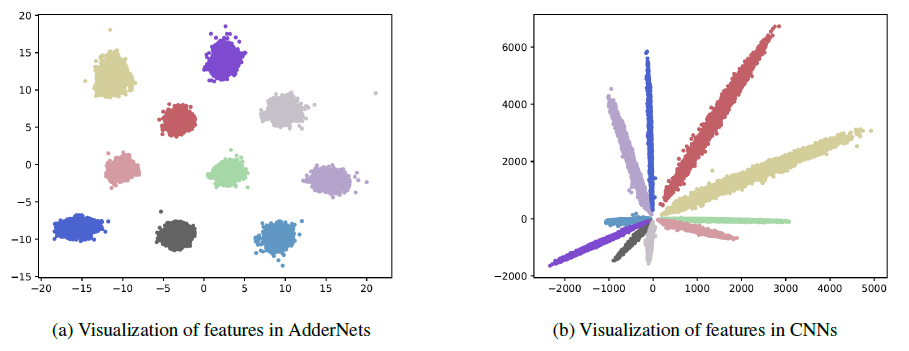Visualization of features in AdderNets and CNNs. Features of CNNs in different classes are divided by their angles.
• A LeNet++ is trained on the MNIST dataset, which has six convolutional layers and a fully-connected layer for extracting powerful 3D features.
• Numbers of neurons in each convolutional layer are 32, 32, 64, 64, 128, 128, and 2, respectively.
• AdderNets utilize the l1-norm to distinguish different classes. The features tend to be clustered towards different class centers.
• The visualization results demonstrate that the proposed AdderNets could have the similar discrimination ability to classify images as CNNs.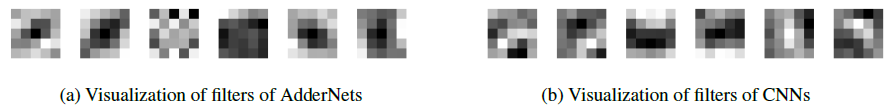Visualization of filters in the first layer of LeNet-5-BN on MNIST
• The filters of the proposed adderNets still share some similar patterns with convolution filters.
• The visualization experiments further demonstrate that the filters of AdderNets can effectively extract useful information from the input images and features.
• The distribution of weights with AdderNets is close to a Laplace distribution while that with CNNs looks more like a Gaussian distribution. In fact, the prior distribution of l1-norm is Laplace distribution.

## 3.5. Ablation Study

• The AdderNets using adaptive learning rate (ALR) and increased learning rate (ILR) achieve 97.99% and 97.72% accuracy with sign gradient, which is much lower than the accuracy of CNN (99.40%).
• Therefore, we propose the full-precision gradient to precisely update the weights in AdderNets.
• As a result, the AdderNet with ILR achieves a 98.99% accuracy using the full-precision gradient. By using the adaptive learning rate (ALR), the AdderNet can achieve a 99.40% accuracy, which demonstrate the effectiveness of the proposed ALR method.

--

--

--

## More from Sik-Ho Tsang

PhD, Researcher. I share what I've learnt and done. :) My LinkedIn: https://www.linkedin.com/in/sh-tsang/, My Paper Reading List: https://bit.ly/33TDhxG

Love podcasts or audiobooks? Learn on the go with our new app.

## Descent carefully on a gradient!## How to Reduce the Technical Debt in ML Projects## Reading: Multi-Scale CNN — Deep Network-Based Frame Extrapolation (HEVC Inter Prediction)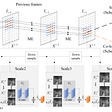## Using Deep Learning to Segment Roads in Aerial Images.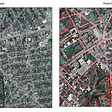## Mathematics for Machine Learning: Linear Algebra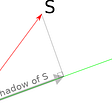## Full Stack Machine Learning on Azure## Object Detection with Keras and Determined## Prelude to Convolutional Neural Networks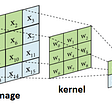## Sik-Ho Tsang

PhD, Researcher. I share what I've learnt and done. :) My LinkedIn: https://www.linkedin.com/in/sh-tsang/, My Paper Reading List: https://bit.ly/33TDhxG

## Review — Multi-task Self-Supervised Visual Learning## Ch 9. Vision Transformer Part I— Introduction and Fine-Tuning in PyTorch## Paper Summary [Deep Deterministic Uncertainty for Semantic Segmentation]## Review — DeiT: Data Efficient Image Transformer